Download the eSaral app and start learning from Kota's top IITians and doctors.

# Tangent & Normal - JEE Main Previous Year Question with Solutions

JEE Main Previous Year Question of Math with Solutions are available at eSaral. Practicing JEE Main Previous Year Papers Questions of mathematics will help the JEE aspirants in realizing the question pattern as well as help in analyzing weak & strong areas. eSaral helps the students in clearing and understanding each topic in a better way. eSaral is providing complete chapter-wise notes of Class 11th and 12th both for all subjects. Besides this, eSaral also offers NCERT Solutions, Previous year questions for JEE Main and Advance, Practice questions, Test Series for JEE Main, JEE Advanced and NEET, Important questions of Physics, Chemistry, Math, and Biology and many more. Download eSaral app for free study material and video tutorials.
Q. The equation of the tangent to the curve $y=x+\frac{4}{x^{2}},$ that is parallel to the x-axis, is :- (1) y = 0                (2) y = 1                 (3) y = 2                    (4) y = 3 [AIEEE-2010]
Ans. (4)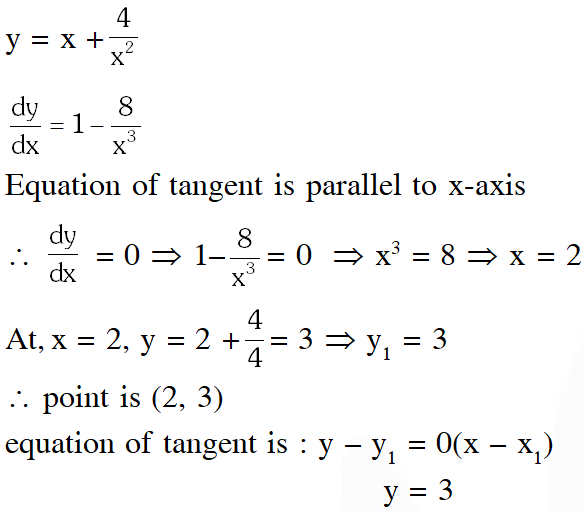Q. The intercepts on x-axis made by tangents to the curve, $\mathrm{y}=\int_{0}^{\mathrm{x}}|\mathrm{t}| \mathrm{dt}, \mathrm{x} \in \mathrm{R}$ which are parallel to the line y = 2x, are equal to $(1) \pm 1$ $(2) \pm 2$ (3) $\pm 3$ (4) $\pm 4$ [JEE-MAIN 2013]
Ans. (1)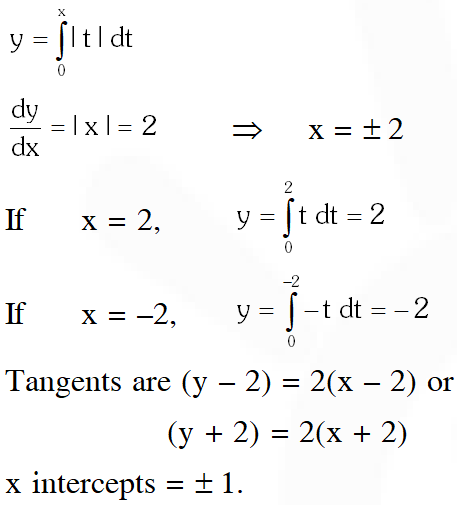Q. The normal to the curve, $x^{2}+2 x y-3 y^{2}=0,$ at $(1,1):$ (1)meets the curve again in the third quadrant (2) meets the curve again in the fourth quadrant (3) does not meet the curve again (4) meets the curve again in the second quadrant [JEE-MAIN 2015]
Ans. (2)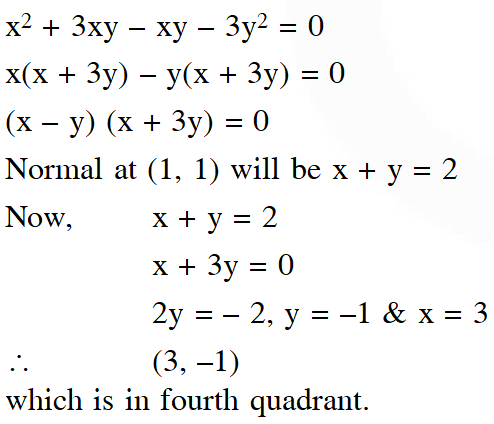Q. Consider $f(x)=\tan ^{-1}(\sqrt{\frac{1+\sin x}{1-\sin x}}), x \in\left(0, \frac{\pi}{2}\right) .$ A normal to $y=f(x)$ at $x=\frac{\pi}{6}$ also passes through the point : ( 1)$\left(\frac{\pi}{4}, 0\right)$b (2) (0, 0) (3) $\left(0, \frac{2 \pi}{3}\right)$ (4) $\left(\frac{\pi}{6}, 0\right)$ [JEE-MAIN 2016]
Ans. (3)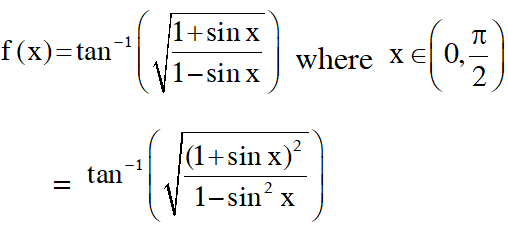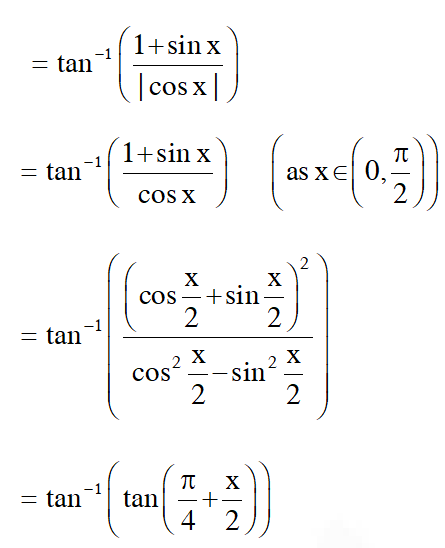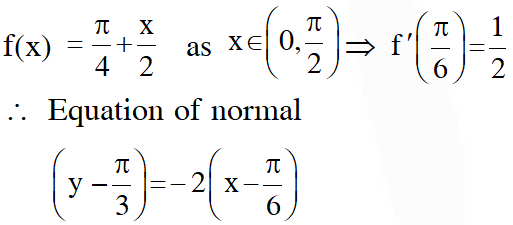Q. If the curves $\mathrm{y}^{2}=6 \mathrm{x}, 9 \mathrm{x}^{2}+\mathrm{by}^{2}=16$ intersect each other at right angles, then the value of $\mathrm{b}$ is : (1) $\frac{7}{2}$             (2) 4              (3) $\frac{9}{2}$                 (4) 6 [JEE-MAIN 2016]
Ans. (3)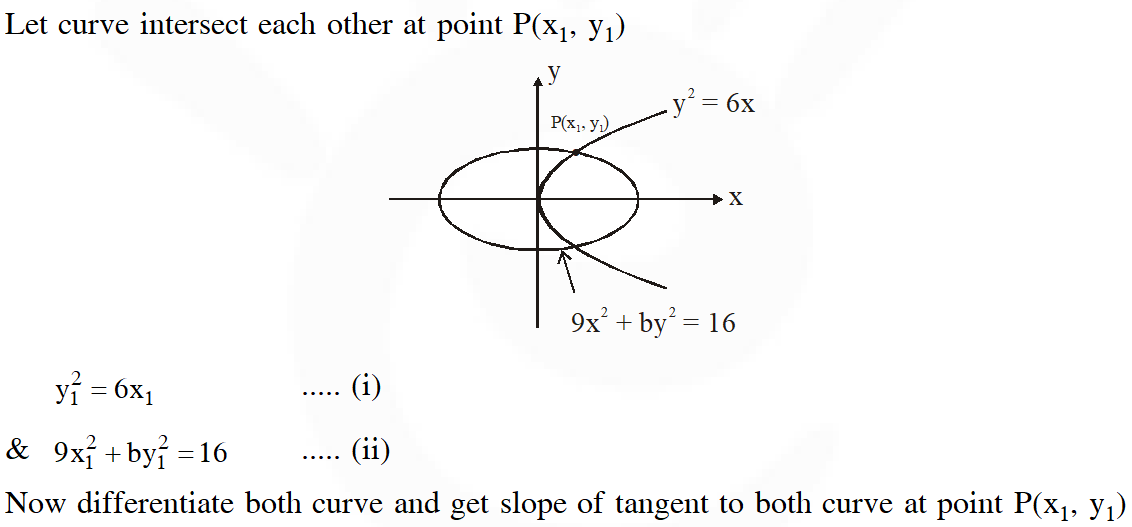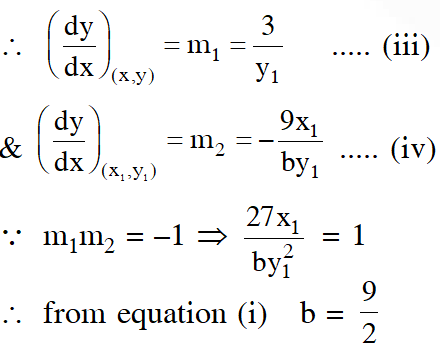Sahil singla
April 15, 2021, 11:12 p.m.
Oh bhai 2016 ke aage bhi badho 2021 ke bhi 2 session ho chuke tum itne pichde kyu ho
Manoj
Dec. 1, 2020, 5:19 p.m.
Op questions
manish
Oct. 29, 2020, 10:19 p.m.
nice
manish
Oct. 20, 2020, 5:05 p.m.
thanx
Anonymous
Oct. 14, 2020, 9:49 p.m.
Good to see all questions here
sumit singh
Oct. 11, 2020, 2:39 p.m.
it is a good platform but no of questions are quite less ...
Soumyakanta Prusty
Sept. 4, 2020, 3:55 p.m.
2 nd last question
Manav
July 21, 2020, 4:25 a.m.
THANKS...FOR ....QUESTIONS
priyanshu dhami
July 10, 2020, 12:08 a.m.
plz can u explain second last question more properly??
vyshnavi b
May 20, 2020, 4:21 p.m.
good but need some more problems..
REHYAA
May 2, 2020, 1:46 p.m.
well gud can have more stuff and content
RIYA
May 2, 2020, 1:46 p.m.
well gud can have more stuff and content
Yoi asshole
April 28, 2020, 5:29 p.m.
Fuck this website
Akhila
April 17, 2020, 3:47 p.m.
Some more
Koushika
April 14, 2020, 5:51 p.m.
Thank you